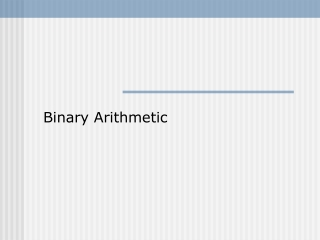Download PresentationBinary Arithmetic

# Binary Arithmetic - PowerPoint PPT PresentationDownload Presentation## Binary Arithmetic

- - - - - - - - - - - - - - - - - - - - - - - - - - - E N D - - - - - - - - - - - - - - - - - - - - - - - - - - -
##### Presentation Transcript

1. Binary Arithmetic

2. Binary Arithmetic • BCD • Binary • Unsigned • 1’s complement • 2’s complement

3. BCD Example-1 Example-2

4. Unsigned - Subtraction The subtraction of two n-digit unsigned numbers M-N(N0) in base r (2) can be done. 1) Add the minuend M to the r’s (2’s) complement of the subtrahend N. This performs M + ( r - N). 2) If M  N , the sum will produce an end carry r which is discarded, and what is the left is is the result M-N.

5. Unsigned - Subtraction (cont.) 3) If M < N , the sum does not produce an end carry and is equal to r - (N - M), which is the r’s complement (2’s) of (N - M). To obtain the answer in a familiar form , take the r’s (2’s) complement of the sum and plant a negative sign in front.

6. Unsigned - Overflow

7. Binary Arithmetic • Two binary numbers can be added, starting at the rightmost bit and adding the corresponding bits in the addend and the augend. • If a carry is generated, it is carried one position to the left, just as in decimal arithmetic. • In 1’s complement arithmetic, a carry generated by the addition of the leftmost bit is added to the rightmost bit. This process is called an end-around carry. • In 2’s complement arithmetic, a carry generated by the addition of the leftmost bits is merely thrown away.

8. Arithmetic with 1’s Complement (8 Bits) Ex1: Ex2: Ex3:

9. Arithmetic with 2’s Complement (8 Bits) • Addition: • Subtraction: • Take the 2’s complement of subtrahend (including the sign bit) and add it to the minuend (including the sign bit). A carry out of the sign bit position is discarded. EX: (+6 )- (-13) = (+6) + (+13) , shown above.

10. Overflow

11. Binary Arithmetic Errors • If the addend and the augend are of opposite signs, overflow error can not occur. • If they are of the same sign and the result is the opposite sign, overflow has occurred and the answer is wrong. • In both 1’s and 2’s complement arithmetic, overflow occurs if and only if the carry into the sign bit differs from the carry out of the sign bit. • Most computers preserve the carry out of the sign bit, but the carry into the sign is not visible from the answer. • For this reason, a special overflow bit is usually provided.

12. Reading : from links • Signed numbers • Floating point numbers